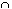Kibiie L.

An experiment consists of two independent trials. The outcomes of the first trial are AB, and C, with probabilities of occurring equal to 0.20.2, and 0.6, respectively. The outcomes of the second trial are E and F, with probabilities of occurring equal to 0.7 and 0.3. Draw a tree diagram representing this experiment. Use this tree diagram to find the probabilities below.

(a)    P(B)

(b)    P(F | B)

(c)    P(BF)

(d)    P(F)